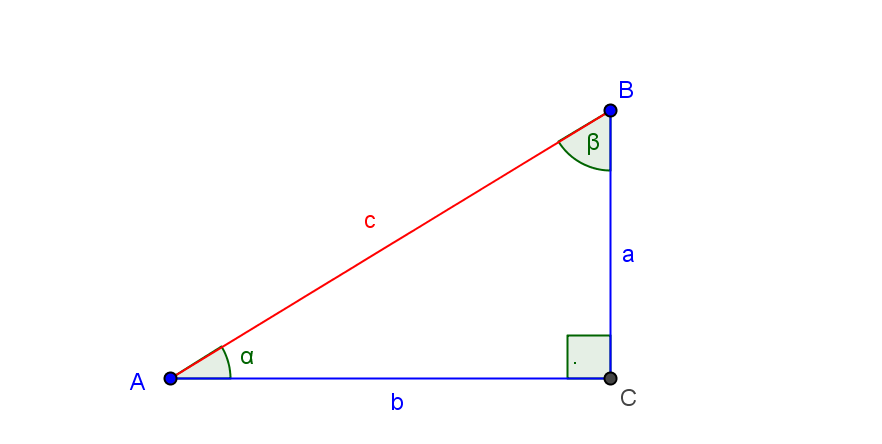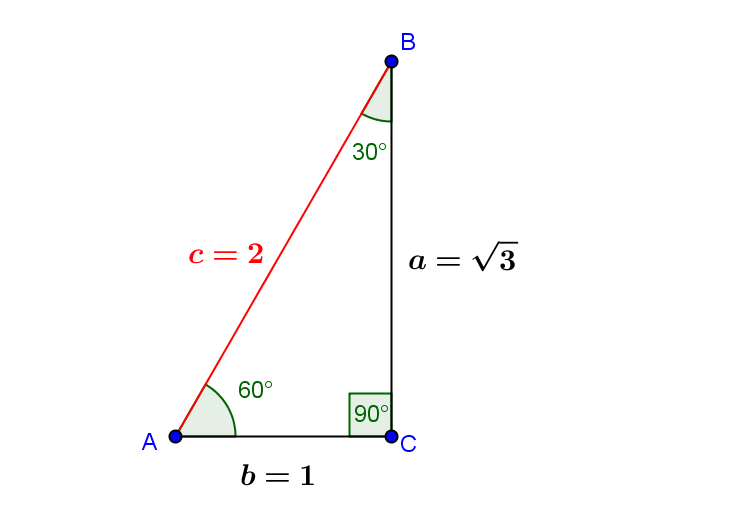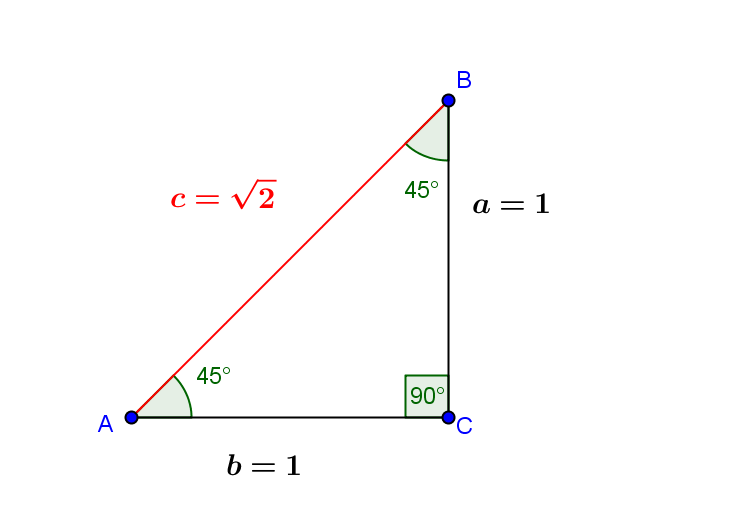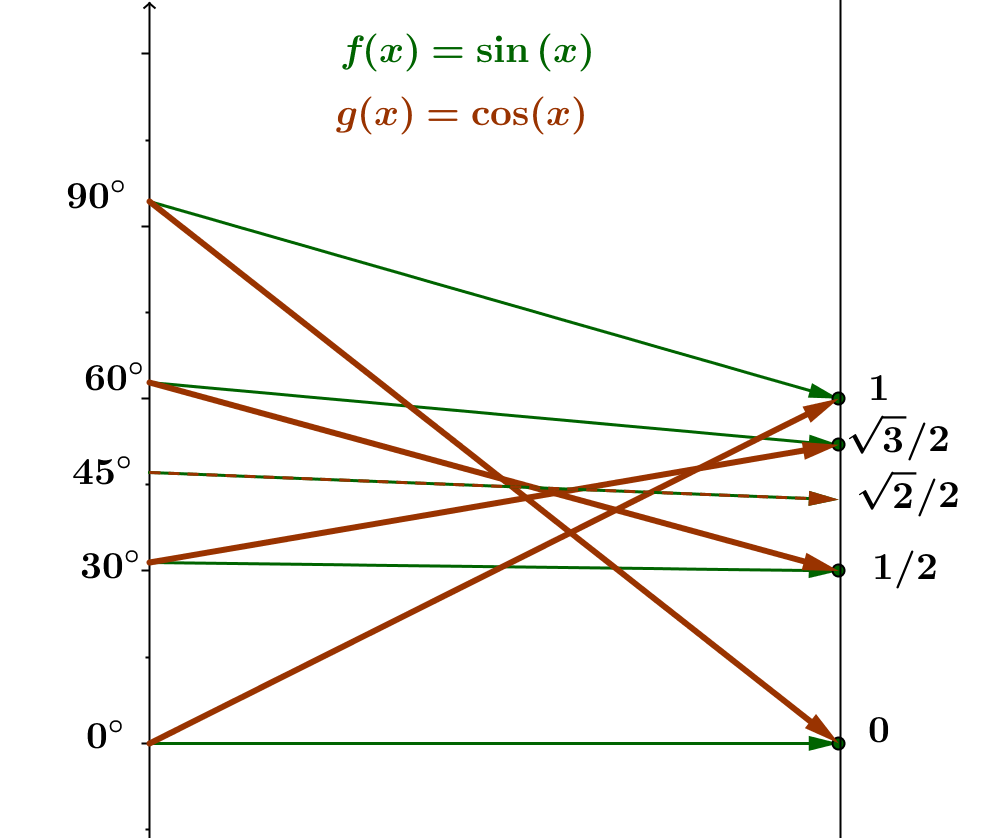(i) Right Triangle Trigonometric Functions
Consider a right triangle $ABC$ with the right angle at vertex $C$, side of length $a$ opposite vertex $A$, with $\angle BAC$  of measure $\alpha$, and side of length $b$ opposite vertex $B$, with $\angle ABC$ of measure $\beta$, and hypotenuse of length $c$.
From the geometry of similar triangles applied to this right triangle, once $\alpha$ is determined, the ratio of any specified pair of sides is constant, depending only on the pair chosen and not the magnitudes of the sides.For $\alpha$ measured in degrees, $0^{\circ} < \alpha < 90^{\circ}$ or $\alpha$  measured with radians, $0 < \alpha < \frac {\pi}2$, based on the choice of sides, the following ratios define the value of the trigonometric function for $\alpha$:

 Function Name Abbreviation for Function Name Function Value for $\alpha$ sine sin $\sin(\alpha) = \frac ac$ cosine cos $\cos(\alpha) = \frac bc$ tangent tan $\tan(\alpha) = \frac ab$ cotangent cot $\cot(\alpha) = \frac ba$ secant sec $\sec(\alpha) = \frac cb$ cosecant csc $\csc(\alpha) = \frac ca$

We start by considering two special triangles, the 30-60-90 and 45-45-90 triangles.Here is a table for the trigonometric functions for the related angles measured in degrees and a simple mapping diagram for the sine and cosine functions also using degree measure:
 $\Theta$ $0^{\circ}$ $30^{\circ}$ $45^{\circ}$ $60^{\circ}$ $90^{\circ}$ $\sin(\Theta)$ $0$ $\frac 12$ $\frac 1{\sqrt{2}} = \frac {\sqrt{2}}2$ $\frac {\sqrt{3}}2$ $1$ $\cos(\Theta)$ $1$ $\frac {\sqrt{3}}2$ $\frac 1{\sqrt{2}} = \frac {\sqrt{2}}2$ $\frac 12$ $0$ $\tan(\Theta)$ $0$ $\frac 1{\sqrt{3}} = \frac {\sqrt{3}}3$ $1$ $\sqrt{3}$ Undefined $\cot(\Theta)$ Undefined $\sqrt{3}$ $1$ $\frac 1{\sqrt{3}} = \frac {\sqrt{3}}3$ $0$ $\sec(\Theta)$ $1$ $\frac 2{\sqrt{3}}= \frac {2\sqrt{3}}3$ $\sqrt{2}$ $2$ Undefined $\csc(\Theta)$ Undefined $2$ $\sqrt{2}$ $\frac 2{\sqrt{3}}= \frac {2\sqrt{3}}3$ $1$Here are two dynamic calculators created with GeoGebra visualizing the triangle trigonometric functions with triangles with $b =1$ (in red) and triangles with $c=1$ (in blue).  The first calculates all six function values while the second focuses on the sine, cosine and tangent functions along with their mapping diagrams using degree measure for the domain axis.